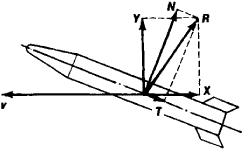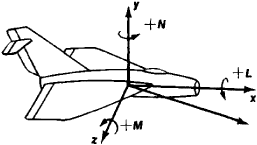# Aerodynamic Force and Moment

The following article is from The Great Soviet Encyclopedia (1979). It might be outdated or ideologically biased.

## Aerodynamic Force and Moment

the quantities that characterize the effect of a gaseous medium on a body—such as an airplane—that is moving in it. The forces of pressure and friction acting on the surface of the body can be reduced to a resultant R of these forces, called the aerodynamic force, and a pair of forces having a moment M, which is called the aerodynamic moment. The aerodynamic force is resolved into components in a rectangular coordinate system (Figure 1) which is associated with either the vectorFigure 1. Resolution of the aerodynamic force into components in the flow coordinate system X, Y, Z and in the correlated system T, N, Z; the Z axis is not shown since it is perpendicular to the plane of the drawing

velocity v of the body (flow or velocity coordinate system) or with the body itself (correlated system). In the flow system the force directed along the axis of flow in a direction opposite to the direction of the body’s motion is called the aerodynamic drag X, the force perpendicular to it and lying in a vertical plane is called the lift Y, and the force perpendicular to both of these is called the side, or lateral, force Z. In the correlated coordinate system the first two forces are, by analogy, the tangential T and normal N forces.

The aerodynamic moment plays an important part in the aerodynamic design of aircraft and in determining their stability and controllability, and it is usually given in the form of three components, which are the projections on the coordinate axes associated with the body (Figure 2): L (rolling moment), N (yawing moment), and M (pitching moment). The values are positive when they tend to turn the body from the y-axis to the z-axis, from the z-axis to the jt-axis, and from the x-axis to the y-axis.Figure 2. Projections of the aerodynamic moment on the coordinate axes: L is the rolling moment, N is the yawing moment, and M is the pitching moment

The aerodynamic forces and moments depend on the shape and dimensions of a body, the speed of its translational motion, its orientation to the direction of the velocity, the properties and the state of the medium in which the motion takes place, and in some cases on the angular rotational velocities and the acceleration of the body’s motion. The determination of aerodynamic forces and moments for bodies of different shapes under all possible flight modes is one of the principal problems of aerodynamics and of aerodynamic experiments.

IU. A. RYZHOV